# Miami-Dade County Public Employee Salary Research – An Analysis in R, Python, and Julia

This analysis was co-authored by data scientists, Scott Fisher and Douglas Davila-Pestana. Mr. Fisher developed the Python code for the analysis, and Mr. Davila-Pestana developed the R and Julia code for the analysis.

# Miami-Dade County Employee Pay: A Comprehensive Data Analysis for Better Transparency

Miami is known for its beaches and its warm weather all year round. There are over 2.6 million people living in sunny Miami-Dade County, Florida (according to the US Census Bureau), and it’s the largest county in Florida in terms of population. It has a two-tier system of government: city and county.  The city government is the first tier, providing local services like police and fire protection, and enforcing city codes, and there are 34 municipalities in Miami-Dade County. Each city, including the largest one, the City of Miami, pays for these services with its own city taxes. The county government is the second tier, handling metropolitan services such as running airports and seaports, emergency management, providing public housing and healthcare, transportation, environmental services, and disposing of solid waste. These services are funded by county taxes.

As such, the county government plays an important role in serving the public, and the Miami-Dade County Government contains numerous departments and employs thousands of employees.

Have you ever wondered how government employee salaries compare across departments, roles, and against national averages? We’ll explore the latest employee pay data from Miami-Dade County for a deep dive into these questions. The dataset we’ll look at is public data from opendata.miamidade.gov which was last updated on February 22, 2023.

Transparency is a cornerstone of republic governance and trust-building between citizens and their government. With this in mind, we delve into this open, public data. Unraveling the details of this data not only increases transparency but also allows us to evaluate the efficiency of how taxpayer dollars are allocated.# A Closer Look at Departments and High Salaries

The dataset comprises salary information from employees of various Miami-Dade County departments. Using this data, we can calculate several statistical summaries by job title and department name, including:

• employee count
• average and median salaries
• salary standard deviation
• salary percentiles
• total sum of department salaries
• department’s ranking by median salary
• department’s ranking by employee count
• Budget Bloat Composite Score Rank – a composite score ranking that signals the department has a high count of employees with high median salaries

Here are a few metrics about Miami-Dade County Government employees based on those summaries:

• Average Salary is \$75,722
• Median Salary is \$69,562
• There are 45 government departments
• There are 29,407 employees
• The total annual salary budget is \$2.22B

For comparison purposes, the median household income of everyone who lives in Miami-Dade County is \$57,815, according to the US Census Bureau. Similar median household income figures can be found from the Federal Reserve Economic Data. And that’s household income, which means it’s the combined income total of everyone living in the household. This means that a single Miami-Dade County government employee has a median salary that is 20% higher than the median household income of residents living in Miami-Dade County.

The top 5 departments with the highest median salaries are the County Attorney’s Office, Independent Civilian Panel, Citizens Independent Transportation Trust, Information Technology, and Inspector General department. These top 5 departments all have a median salary of \$120,000 or higher. 10 out of the 45 government departments have a median salary of \$100,000 or more. You can view the median salary by department in the chart below: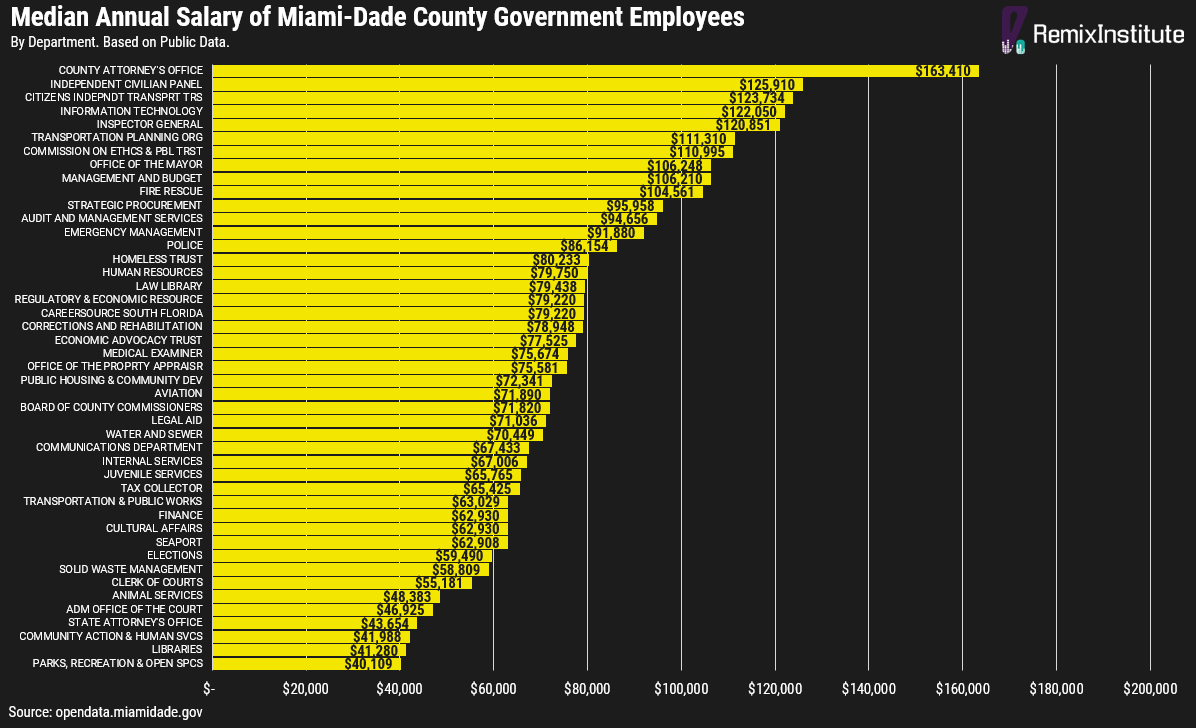There’s only a few departments of the Miami Dade-County government which make up the majority of the annual salary budget. The top 2 departments which make up the largest percent of the annual salary budget are the Police department and Fire Rescue department, which combined comprise almost 1/3 of the total annual salary budget (30%). The top 6 departments comprise almost 2/3 of the total annual salary budget (64%): Police, Fire Rescue, Transportation and Public Works, Corrections and Rehabilitation, Water and Sewer, and Aviation.Departments with high count of employees and with high median salaries are going to have the highest salary budget bloat. That’s where we calculated the Budget Bloat Composite Score Rank that was discussed previously in the article. Based on the Budget Bloat Composite Score Rank, the top 5 departments with the highest salary budget bloat are: Fire Rescue, Police, Information Technology, Corrections and Rehabilitation, and County Attorney’s Office.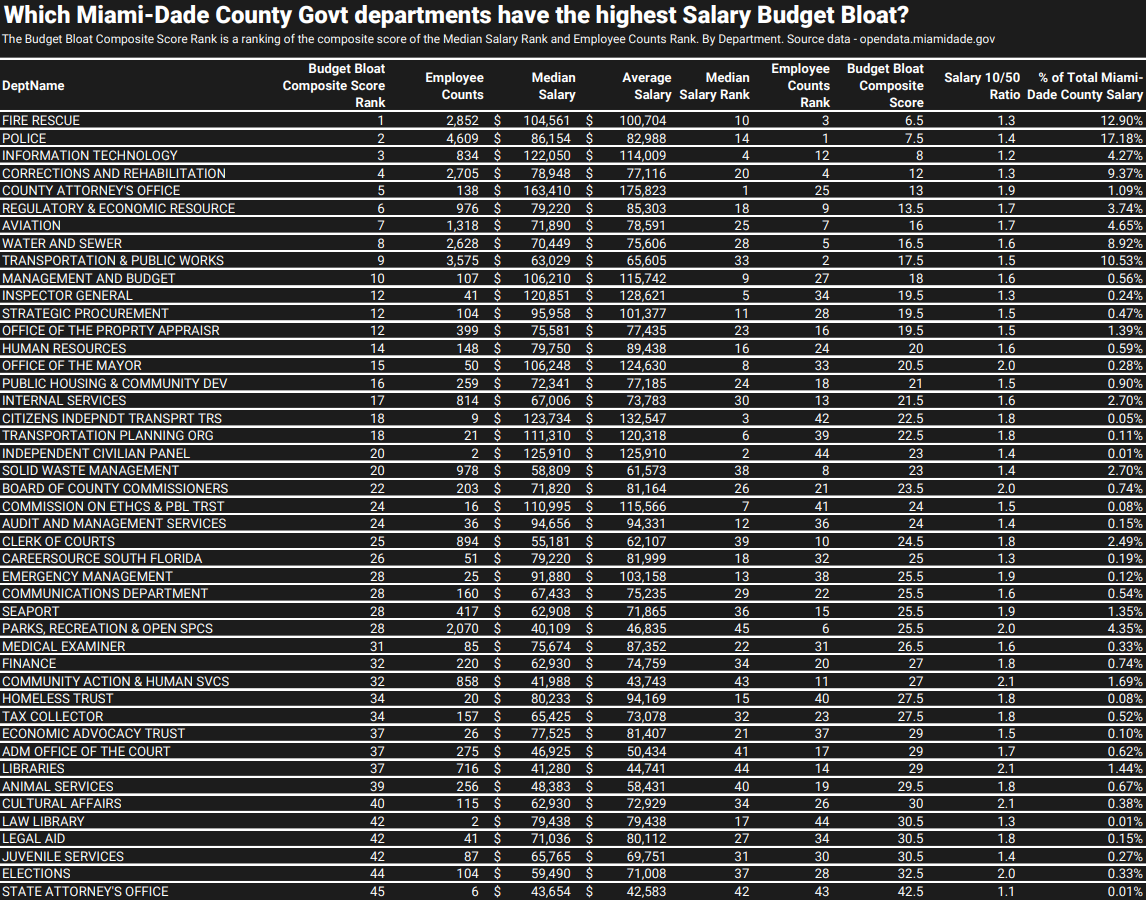# The \$100K Club

We also analyzed how many government employees for Miami-Dade County make over \$100,000 annually. The figure stands at 6,598, representing 22% of the total 29,407 Miami-Dade County government employees. This shows that while a six-figure salary is not uncommon, it’s only a reality for a minority of employees.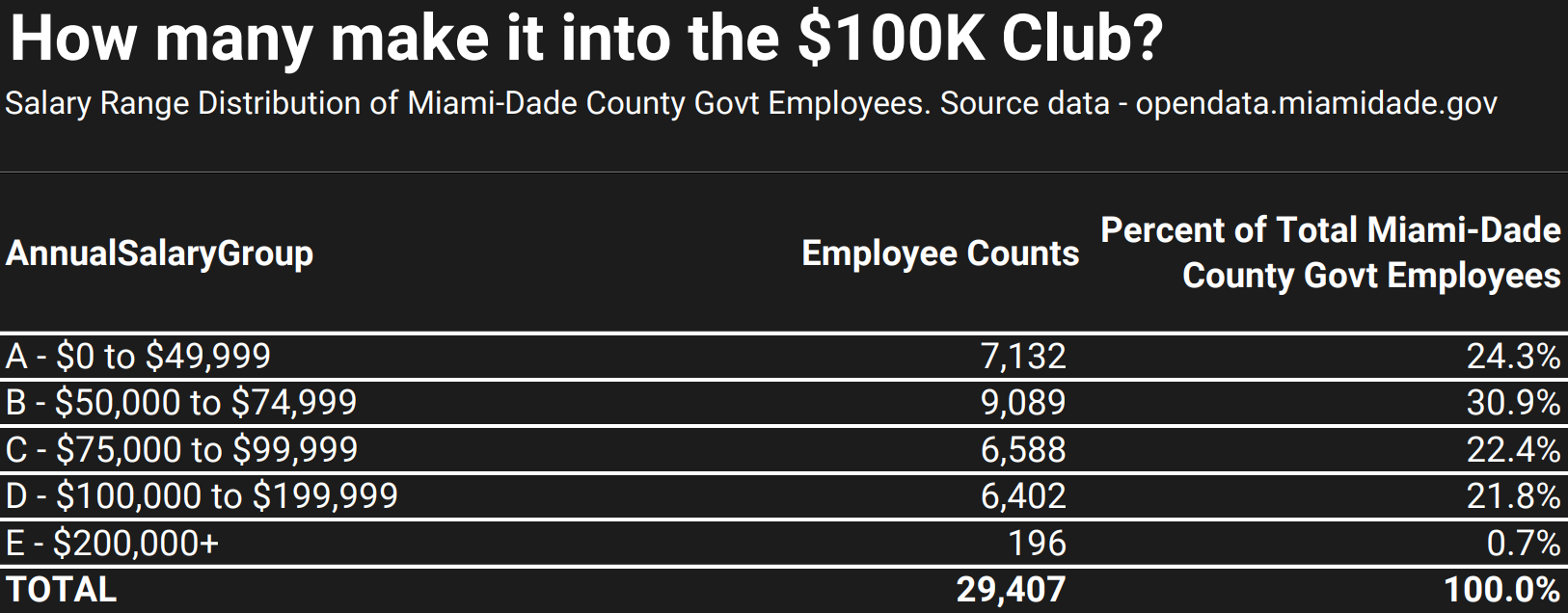# Top Paid Positions

We also examined the highest-paid roles across the Miami-Dade County government. Of the total unique 3,290 job positions, the top 96 positions emerged as having the highest median salaries of \$200,000 or more per year. These lucrative roles are not confined to specific departments. The table below shows the top 40 annual median salaries by title and department name.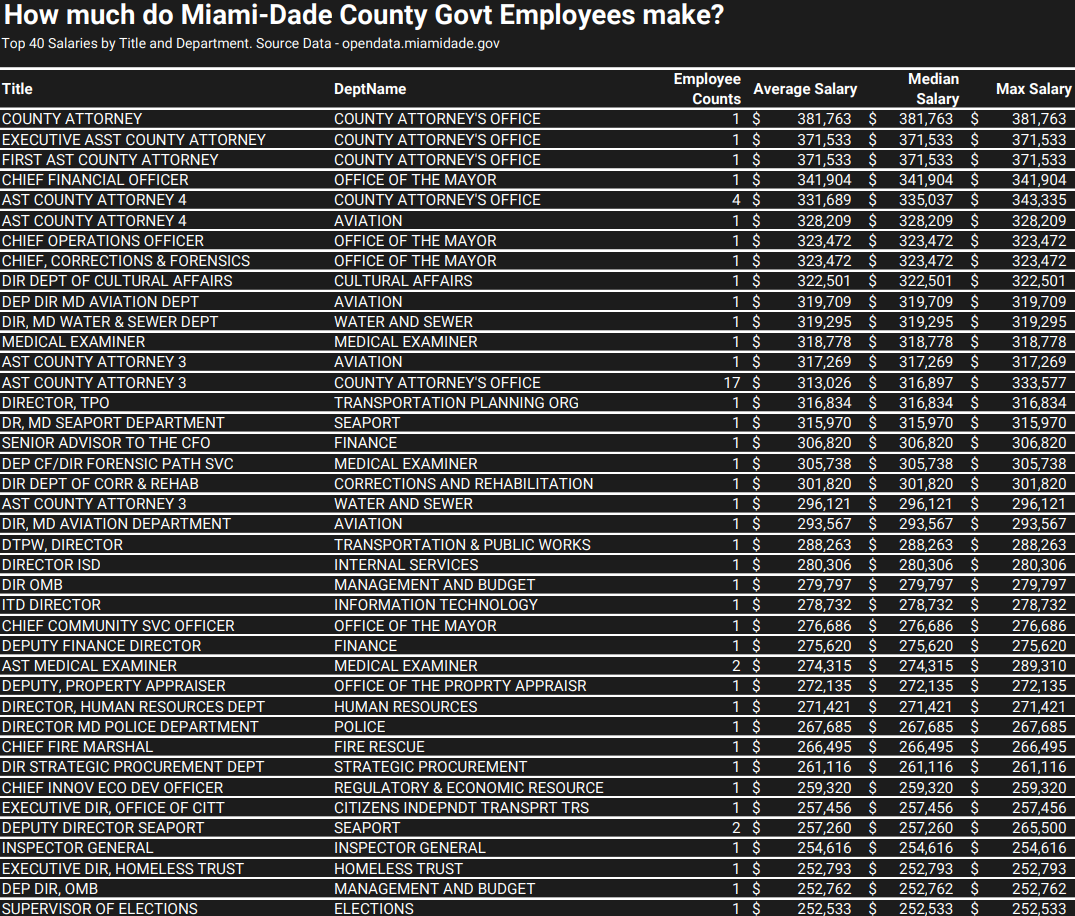# Average Salaries of Data and Analyst Positions

Data and analyst roles have been garnering increased attention in the media due to their pivotal role in driving insights, supporting optimal decision-making, and developing AI-based solutions. Our analysis found that these roles earn an average of \$91,126 as an employee in the Miami-Dade County government. The table below shows the top 40 annual median salaries by department for job titles with the word “data” or “analyst” in its name.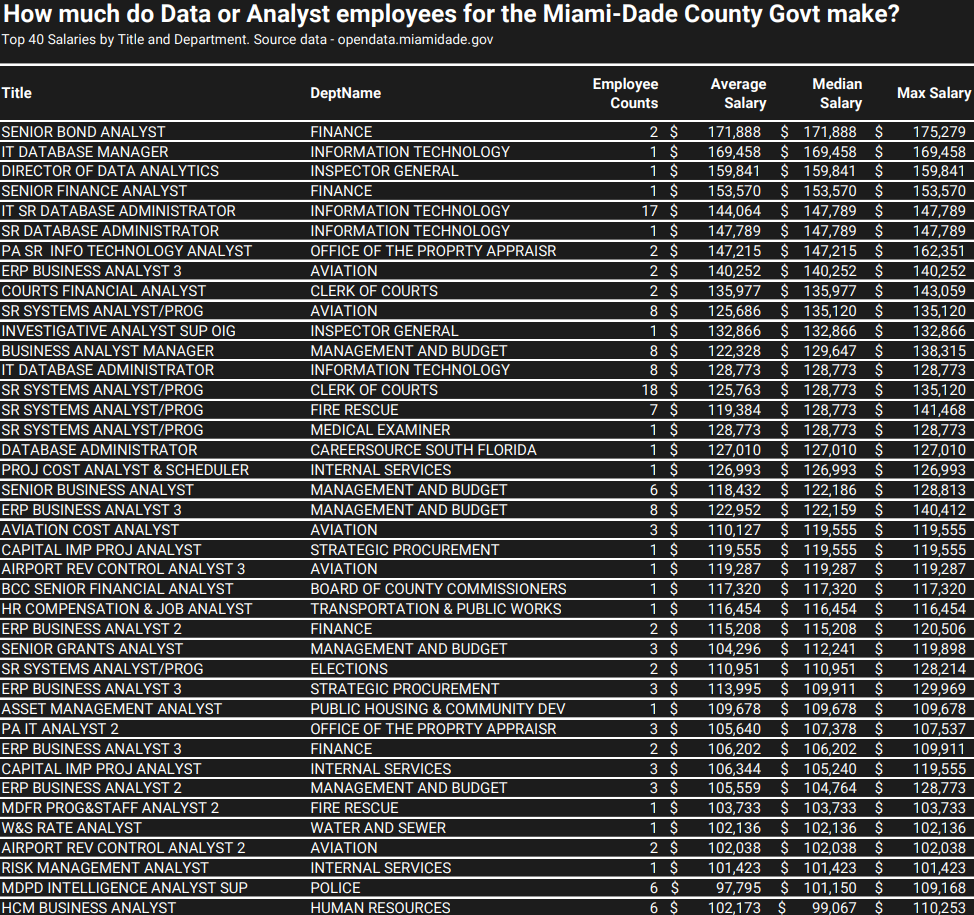# Salary Comparisons with National, State, and City Averages

Comparing the salaries within the Miami-Dade County government to overall national, state, and city averages offers a broader perspective.

For instance, the average attorney working for the Miami-Dade County government earns \$219,297, which is over \$100,000 higher than the Miami city average from Payscale of \$91,573 and the Miami city average from Glassdoor of \$103, 515.

Similarly, the average Police Officer working for the Miami-Dade County government earns \$90,098, if you look at the table by Title and Department above. This is over \$15,000 higher than both the Florida state and national average Police Officer salaries of \$73,350 and \$71,380 respectively, according the US Bureau of Labor Statistics.

The average Firefighter working for the Miami-Dade County government earns \$96,744, if you look at the table by Title and Department above. This is over \$40,000 higher than both the Florida state and national average Firefighter salaries of \$56,560 and \$56,310 respectively, according to the US Bureau of Labor Statistics.

It’s worth noting that some of these salaries, particularly within the police and fire rescue departments, may be higher due to the influence of labor unions such as the South Florida Police Benevolent Association (PBA) and the Metro-Dade Firefighters Local 1403. These unions often negotiate for better pay, benefits, and working conditions for their members. In fact, the South Florida PBA represents the following units:  Miami-Dade Police Department, Miami-Dade County Department of Corrections and Rehabilitation, Commission on Ethics and Public Trust Employees, and Miami-Dade Animal Services. The presence of police and firefighter unions in Miami-Dade County could contribute to higher-than-average salaries for these roles, a factor that is important to consider when comparing salaries across different regions and sectors.

# “Peasant” Labor: Income Inequality Within Departments

An intriguing facet of our analysis is the income inequality metrics we calculated: the 20/20 ratio, the Palma ratio, the 10/50 ratio, and the Coefficient of Variation.

Let’s break down these inequality metrics in layman’s terms:

•  20/20 Ratio: This metric compares the top 20th percentile salary to the bottom 20th percentile salary within a department. If the 20/20 ratio is significantly greater than 1, it suggests that higher earners in the department make much more than the lower earners, indicating wage disparity.
• Palma Ratio: Similar to the 20/20 Ratio, the Palma Ratio compares the top 10th percentile salary to the bottom 40th percentile. This metric focuses more on the disparity between the very top earners and the middle band.
• 10/50 Ratio: Similar to both the 20/20 Ratio and the Palma Ratio, this metric compares the top 10th percentile salary to the bottom 50th percentile salary within a department. If the 10/50 ratio is significantly greater than 1, it suggests that very top earners in the department make much more than the median earner, indicating significant wage disparity. The 10/50 Ratio is what is displayed in the chart below.
• Coefficient of Variation (CV): The CV is the ratio of the standard deviation of the salaries to their mean. A higher CV indicates more significant disparity in the salary range, while a lower CV shows more uniformity.

These metrics provide a robust picture of income distribution within each department, highlighting areas of wage disparity that may warrant further investigation. The departments towards the top of the list may mean that the bottom 50th percentile of employees there may be treated as peons or “peasant” labor. The top 5 departments with the highest income inequality among employees in that department are: Cultural Affairs, Board of County Commissioners, Elections, Office of the Mayor, and the County Attorney’s Office. In these departments, the top 10% of earners make about 2x more than the bottom 50% of earners.# Concluding Thoughts

In conclusion, delving into public salary data reveals vital insights about income distribution, wage disparity, and overall payroll spending within the Miami-Dade County government. Increased transparency and careful analysis of these data points can lead to better accountability and more effective use of taxpayer dollars. Ensuring equitable wage distribution and efficient allocation of public funds is an ongoing process, and openness of data plays a crucial role in facilitating this endeavor.

# R Code

```				```
# start time
start_time = Sys.time()

# Load required data preparation libraries
library(data.table)
library(dplyr)
library(magrittr)
library(ggplot2)
library(extrafont)
library(scales)
library(grid)
library(magick)

# Dataset name: "Employee Pay Information"

# IMPORT EMPLOYEE PAY DATA FROM MIAMI DADE COUNTY --------

employee_dataframe\$AnnualSalaryGroup = ifelse(employee_dataframe\$AnnualSalary < 50000, "A - \$0 to \$49,999",
ifelse(employee_dataframe\$AnnualSalary < 75000, "B - \$50,000 to \$74,999",
ifelse(employee_dataframe\$AnnualSalary < 100000, "C - \$75,000 to \$99,999",
ifelse(employee_dataframe\$AnnualSalary < 200000, "D - \$100,000 to \$199,999",
ifelse(employee_dataframe\$AnnualSalary >= 200000, "E - \$200,000+", "F - Unknown")))))
# what is the average salary?
print(paste0("The average salary of Miami-Dade County government employees is: ", mean(employee_dataframe\$AnnualSalary, na.rm = TRUE) %>% round(0)))
# what is the median salary?
print(paste0("The median salary of Miami-Dade County government employees is: ", median(employee_dataframe\$AnnualSalary, na.rm = TRUE) %>% round(0)))
# how many employees?
print(paste0("The total number of Miami-Dade County government employees is: ", nrow(employee_dataframe)))
# what is the total salary?
print(paste0("The total salary of Miami-Dade County government employees is: ", sum(employee_dataframe\$AnnualSalary, na.rm = TRUE) %>% round(2)))

# compare median salary of miami dade county govt employees to entire labor force in miami-dade county
# Federal Reserve Economic Data: https://fred.stlouisfed.org/series/MHIFL12086A052NCEN

# DATA PREPARATION AND ANALYSIS ------

# percentile distributions - total and by dept

# summary by dept
employee_dataframe_dept_summ = employee_dataframe %>% dplyr::group_by(DeptName) %>%
dplyr::summarize(Employee_Counts = n(),
Avg_Salary = mean(AnnualSalary, na.rm = TRUE),
Median_Salary = median(AnnualSalary, na.rm = TRUE),
StdDev_Salary = sd(AnnualSalary, na.rm = TRUE),
Salary_CoefficientOfVariation = sd(AnnualSalary, na.rm = TRUE) / mean(AnnualSalary, na.rm = TRUE),
Salary_20_20_Ratio = (quantile(AnnualSalary, c(.80)) / quantile(AnnualSalary, c(.20))) %>% as.numeric(), # https://en.wikipedia.org/wiki/Income_inequality_metrics
Salary_Palma_Ratio = (quantile(AnnualSalary, c(.90)) / quantile(AnnualSalary, c(.40))) %>% as.numeric(), # https://en.wikipedia.org/wiki/Income_inequality_metrics
Salary_10_50_Ratio = (quantile(AnnualSalary, c(.90)) / quantile(AnnualSalary, c(.50))) %>% as.numeric(),
Min_Salary = min(AnnualSalary, na.rm = TRUE),
Salary_20thPercentile = quantile(AnnualSalary, c(.20)) %>% as.numeric(),
Salary_40thPercentile = quantile(AnnualSalary, c(.40)) %>% as.numeric(),
Salary_80thPercentile = quantile(AnnualSalary, c(.80)) %>% as.numeric(),
Salary_90thPercentile = quantile(AnnualSalary, c(.90)) %>% as.numeric(),
Max_Salary = max(AnnualSalary, na.rm = TRUE),
Sum_Salary = sum(AnnualSalary, na.rm = TRUE)
)
# sort it by median salary
employee_dataframe_dept_summ = employee_dataframe_dept_summ[order(employee_dataframe_dept_summ\$Median_Salary, decreasing = TRUE), ]
employee_dataframe_dept_summ\$Pct_Of_TotalMDCounty_Salary = round(employee_dataframe_dept_summ\$Sum_Salary / sum(employee_dataframe_dept_summ\$Sum_Salary, na.rm = TRUE), 4)
employee_dataframe_dept_summ\$Pct_Of_TotalMDCounty_Employees = round(employee_dataframe_dept_summ\$Employee_Counts / sum(employee_dataframe_dept_summ\$Employee_Counts, na.rm = TRUE), 4)
# add a ranking of Median_Salary and Employee_Counts in descending order
employee_dataframe_dept_summ\$Median_Salary_Rank = data.table::frank(-employee_dataframe_dept_summ\$Median_Salary) %>% round(0)
employee_dataframe_dept_summ\$Employee_Counts_Rank = data.table::frank(-employee_dataframe_dept_summ\$Employee_Counts) %>% round(0)
# created a Composite Score by taking an average of the Median_Salary_Rank and Employee_Counts_Rank: a higher ranking represents a more bloated budget
employee_dataframe_dept_summ\$Budget_Bloat_Composite_Score = rowMeans(cbind(employee_dataframe_dept_summ\$Median_Salary_Rank, employee_dataframe_dept_summ\$Employee_Counts_Rank), na.rm = TRUE) %>% round(1)
employee_dataframe_dept_summ\$Budget_Bloat_Composite_Score_Rank = data.table::frank(employee_dataframe_dept_summ\$Budget_Bloat_Composite_Score) %>% round(0)
# export to csv

# export to csv
employee_dataframe_dept_summ_subset = subset(employee_dataframe_dept_summ, select = c(
DeptName,
Budget_Bloat_Composite_Score_Rank,
Employee_Counts,
Median_Salary,
Avg_Salary,
Median_Salary_Rank,
Employee_Counts_Rank,
Budget_Bloat_Composite_Score,
Salary_10_50_Ratio,
Pct_Of_TotalMDCounty_Salary
)) %>%
dplyr::rename(
`Budget Bloat Composite Score Rank` =  Budget_Bloat_Composite_Score_Rank,
`Employee Counts` = Employee_Counts,
`Median Salary` = Median_Salary,
`Average Salary` = Avg_Salary,
`Median Salary Rank` = Median_Salary_Rank,
`Employee Counts Rank` = Employee_Counts_Rank,
`Budget Bloat Composite Score` = Budget_Bloat_Composite_Score,
`Salary 10/50 Ratio` = Salary_10_50_Ratio,
`% of Total Miami-Dade County Salary` = Pct_Of_TotalMDCounty_Salary
)
# sort by Budget Bloat Composite Score Rank
employee_dataframe_dept_summ_subset = employee_dataframe_dept_summ_subset[order(employee_dataframe_dept_summ_subset\$`Budget Bloat Composite Score Rank`, decreasing = FALSE), ]

# how many are employees make over \$100K
employee_dataframe_gte100K = subset(employee_dataframe, AnnualSalary >= 100000)
print(paste0(nrow(employee_dataframe_gte100K), " of ", nrow(employee_dataframe), " Miami-Dade County government employees make over \$100K in salary."))
# summary of employee salary ranges
employee_dataframe_salary_range_summ = employee_dataframe %>% dplyr::group_by(AnnualSalaryGroup) %>%
dplyr::summarize(`Employee Counts` = n())
employee_dataframe_salary_range_summ\$`Percent of Total Miami-Dade County Govt Employees` = round(employee_dataframe_salary_range_summ\$`Employee Counts` / sum(employee_dataframe_salary_range_summ\$`Employee Counts`, na.rm = TRUE), 4)
employee_dataframe_salary_range_summ[nrow(employee_dataframe_salary_range_summ) + 1, ] = cbind(NA, NA, NA)
employee_dataframe_salary_range_summ[nrow(employee_dataframe_salary_range_summ), "AnnualSalaryGroup"] = "TOTAL"
employee_dataframe_salary_range_summ[nrow(employee_dataframe_salary_range_summ), "Employee Counts"] = sum(employee_dataframe_salary_range_summ\$`Employee Counts`, na.rm = TRUE)
employee_dataframe_salary_range_summ[nrow(employee_dataframe_salary_range_summ), "Percent of Total Miami-Dade County Govt Employees"] = sum(employee_dataframe_salary_range_summ\$`Percent of Total Miami-Dade County Govt Employees`, na.rm = TRUE)

# export to csv

# top paid positions

# summary by title and dept
employee_dataframe_title_and_dept_summ = employee_dataframe %>% dplyr::group_by(Title, DeptName) %>%
dplyr::summarize(`Employee Counts` = n(),
`Average Salary` = mean(AnnualSalary, na.rm = TRUE),
`Median Salary` = median(AnnualSalary, na.rm = TRUE),
`Max Salary` = max(AnnualSalary, na.rm = TRUE),
)
# sort it by median salary
employee_dataframe_title_and_dept_summ = employee_dataframe_title_and_dept_summ[order(employee_dataframe_title_and_dept_summ\$`Median Salary`, decreasing = TRUE), ]

# export to csv

# compare with average lawyers salary in miami

# Miami (Payscale): https://www.payscale.com/research/US/Job=Attorney_%2F_Lawyer/Salary/b37de149/Miami-FL
# Miami (Glassdoor): https://www.glassdoor.com/Salaries/miami-lawyer-salary-SRCH_IL.0,5_IC1154170_KO6,12.htm
employee_dataframe_lawyer = subset(employee_dataframe, tolower(Title) %like% 'attorney')
# average salary of attorney positions
print(paste0("The average salary of of an attorney in Miami-Dade County govt is: ", mean(employee_dataframe_lawyer\$AnnualSalary, na.rm = TRUE) %>% round(0)))

# compare with average policy salary in the us and florida
# https://www.bls.gov/oes/current/oes333051.htm

# compare with average firefighter salary in the us and florida
# https://www.bls.gov/oes/current/oes332011.htm

# find "DATA" or "ANALYST" positions and see how much they're paid.
employee_dataframe_data_and_analyst_jobs = subset(employee_dataframe_title_and_dept_summ, tolower(Title) %like% 'analyst' | tolower(Title) %like% 'data')
employee_dataframe_data_and_analyst = subset(employee_dataframe, tolower(Title) %like% 'analyst' | tolower(Title) %like% 'data')
# average salary of "DATA" or "ANALYST" positions
print(paste0("The average salary of a data or analyst employee in Miami-Dade County govt is: ", mean(employee_dataframe_data_and_analyst\$AnnualSalary, na.rm = TRUE) %>% round(0)))

# export to csv

# end time
end_time = Sys.time()

# how long did it take
duration = end_time - start_time
print("The process took ")
print(duration)

# DATA VISUALIZATION CHARTS -----------

# Font Import
extrafont::font_import(pattern = "Roboto")
y

# Sorted Bar Chart for Median Salaries by Department
median_salary_plot = ggplot2::ggplot(employee_dataframe_dept_summ, aes(reorder(DeptName, Median_Salary), Median_Salary)) +
geom_bar(stat = 'identity', fill = '#F3E600') +
geom_text(aes(label=scales::dollar(Median_Salary)), hjust=1, color="black", fontface = "bold") +
geom_text(aes(label=DeptName), hjust=-0.1, color="white", fontface = "bold") +
coord_flip() +
scale_y_continuous(labels = scales::dollar, expand = expansion(mult = c(0, 0.1)), limits = c(0, 200000)) + # set max limit of Median_Salary axis to 200,000
labs(title = "Median Annual Salary of Miami-Dade County Government Employees",
subtitle = "By Department. Based on Public Data.",
theme_minimal() +
theme(plot.background = element_rect(fill = '#1c1c1c'),
plot.margin = margin(5.5, 5.5, 5.5, 10, "pt"), # add a 10pt margin to the left of the plot
text = element_text(family = "Roboto", color = "white"),
axis.text.y = element_blank(),  # remove DeptName axis text
axis.text.x = element_text(color = "white", size = 14),  # increase Median_Salary axis text font size
plot.title = element_text(size = 20, hjust = 0, face = "bold"),
plot.subtitle = element_text(size = 12, hjust = 0),
plot.caption = element_text(size = 12, hjust = 0),  # increase caption font size
axis.title = element_blank(),
panel.grid.minor = element_blank(),
panel.grid.major.y = element_blank(),
axis.ticks.y = element_blank())

# Remix Institute branding
median_salary_plot
grid::grid.raster(logo, x = .87, y = .93, just = c('left', 'bottom'), width = 0.12)

# Sorted Bar Chart for Total Miami-Dade County Salary Consumption by Department
salary_percentage_plot = ggplot2::ggplot(employee_dataframe_dept_summ, aes(reorder(DeptName, Pct_Of_TotalMDCounty_Salary), Pct_Of_TotalMDCounty_Salary*100)) +
geom_bar(stat = 'identity', fill = '#00aa9d') +
geom_text(aes(label=sprintf("%.2f%%", Pct_Of_TotalMDCounty_Salary*100)), hjust=1, color="white", fontface = "bold") +
#geom_text(aes(label=DeptName), hjust=-0.1, color="white", fontface = "bold") +
coord_flip() +
scale_y_continuous(expand = expansion(mult = c(0.03, 0.2))) + # 3% space below the bars but 20% above them
labs(title = "Which Miami-Dade County Government Employees Have The Highest Percentage\nof the Annual Salary Budget?",
subtitle = "Total Annual Salary Distribution by Department. Based on Public Data",
theme_minimal() +
theme(plot.background = element_rect(fill = '#1c1c1c'),
plot.margin = margin(5.5, 5.5, 5.5, 10, "pt"), # add a 10pt margin to the left of the plot
text = element_text(family = "Roboto", color = "white"),
#axis.text.y = element_blank(),  # remove DeptName axis text
axis.text.y = element_text(color = "white"),
axis.text.x = element_text(color = "white", size = 14),  # increase Pct_Of_TotalMDCounty_Salary axis text font size
plot.title = element_text(size = 20, hjust = 0, face = "bold"),
plot.subtitle = element_text(size = 12, hjust = 0),
plot.caption = element_text(size = 12, hjust = 0), # increase caption font size
axis.title = element_blank(),
panel.grid.minor = element_blank(),
panel.grid.major.y = element_blank(),
axis.ticks.y = element_blank())

# Remix Institute branding
salary_percentage_plot
grid::grid.raster(logo, x = .87, y = .93, just = c('left', 'bottom'), width = 0.12)

# Sorted Bar Chart for Miami-Dade County Salary Income Inequality by Department
income_inequality_plot = ggplot2::ggplot(subset(employee_dataframe_dept_summ, Median_Salary >= 50000 & Employee_Counts >= 10), aes(reorder(DeptName, Salary_10_50_Ratio), Salary_10_50_Ratio)) +
geom_bar(stat = 'identity', fill = '#ed2590') +
geom_text(aes(label = paste0(round(Salary_10_50_Ratio,1),"x")), hjust=1, color="white", fontface = "bold") +
#geom_text(aes(label=DeptName), hjust=-0.1, color="white", fontface = "bold") +
coord_flip() +
scale_y_continuous(expand = expansion(mult = c(0.02, 0.2)), limits = c(1, 3), oob = scales::squish) + # 2% space below the bars but 20% above them, limit from 1 to 4
labs(title = "Miami-Dade County Government Departments with Highest Income Inequalities",
subtitle = "What the top 10% Annual Salary Earners make versus the bottom 50% Annual Salary Earners. For Departments\nwith Median Annual Salary >= \$50K and More Than 10 Employees. Based on Public Data.",
theme_minimal() +
theme(plot.background = element_rect(fill = '#1c1c1c'),
plot.margin = margin(5.5, 5.5, 5.5, 10, "pt"), # add a 10pt margin to the left of the plot
text = element_text(family = "Roboto", color = "white"),
axis.text.y = element_text(color = "white"),
axis.text.x = element_text(color = "white", size = 14),  # increase Salary_10_50_Ratio axis text font size
plot.title = element_text(size = 20, hjust = 0, face = "bold"),
plot.subtitle = element_text(size = 12, hjust = 0),
plot.caption = element_text(size = 12, hjust = 0), # increase caption font size
axis.title = element_blank(),
panel.grid.minor = element_blank(),
panel.grid.major.y = element_blank(),
axis.ticks.y = element_blank())

# Remix Institute branding
income_inequality_plot
grid::grid.raster(logo, x = .87, y = .93, just = c('left', 'bottom'), width = 0.12)

```
```

# Julia Code

```				```
# start time
using Dates
start_time = now()

# Load required data preparation packages
using CSV
using DataFrames
using Statistics
using Printf
using Missings
using DataFramesMeta
using Pipe
using Glob
using Chain

# Dataset name: "Employee Pay Information"

# IMPORT EMPLOYEE PAY DATA FROM MIAMI DADE COUNTY --------

employee_dataframe.AnnualSalaryGroup = ifelse.(employee_dataframe.AnnualSalary .< 50000, "A - \\$0 to \\$49,999",
ifelse.(employee_dataframe.AnnualSalary .< 75000, "B - \\$50,000 to \\$74,999",
ifelse.(employee_dataframe.AnnualSalary .< 100000, "C - \\$75,000 to \\$99,999",
ifelse.(employee_dataframe.AnnualSalary .< 200000, "D - \\$100,000 to \\$199,999",
ifelse.(employee_dataframe.AnnualSalary .>= 200000, "E - \\$200,000+", "F - Unknown")))))

# What is the average salary?
println("The average salary of Miami-Dade County government employees is: ", @sprintf("%.0f", mean(skipmissing(employee_dataframe.AnnualSalary))))

# What is the median salary?
println("The median salary of Miami-Dade County government employees is: ", @sprintf("%.0f", median(skipmissing(employee_dataframe.AnnualSalary))))

# How many employees?
println("The total number of Miami-Dade County government employees is: ", nrow(employee_dataframe))

# What is the total salary?
println("The total salary of Miami-Dade County government employees is: ", @sprintf("%.2f", sum(skipmissing(employee_dataframe.AnnualSalary))))

# Compare median salary of Miami Dade County govt employees to entire labor force in Miami-Dade County
# Federal Reserve Economic Data: https://fred.stlouisfed.org/series/MHIFL12086A052NCEN

# DATA PREPARATION AND ANALYSIS ------

# custom rank function
function rank(v)
n = length(v)
r = sortperm(v)
r_inv = Array{Int64}(undef, n)
r_inv[r] = 1:n
return r_inv
end

# percentile distributions - total and by dept

# Summary by Dept
group_by = groupby(employee_dataframe, :DeptName)
employee_dataframe_dept_summ = combine(group_by,
:AnnualSalary => (x -> count(!ismissing, x)) => :Employee_Counts,
:AnnualSalary => (x -> mean(skipmissing(x))) => :Avg_Salary,
:AnnualSalary => (x -> median(skipmissing(x))) => :Median_Salary,
:AnnualSalary => (x -> std(skipmissing(x))) => :StdDev_Salary,
:AnnualSalary => (x -> std(skipmissing(x)) / mean(skipmissing(x))) => :Salary_CoefficientOfVariation,
:AnnualSalary => (x -> quantile(skipmissing(x), 0.8) / quantile(skipmissing(x), 0.2)) => :Salary_20_20_Ratio,
:AnnualSalary => (x -> quantile(skipmissing(x), 0.9) / quantile(skipmissing(x), 0.4)) => :Salary_Palma_Ratio,
:AnnualSalary => (x -> quantile(skipmissing(x), 0.9) / quantile(skipmissing(x), 0.5)) => :Salary_10_50_Ratio,
:AnnualSalary => (x -> minimum(skipmissing(x))) => :Min_Salary,
:AnnualSalary => (x -> quantile(skipmissing(x), 0.2)) => :Salary_20thPercentile,
:AnnualSalary => (x -> quantile(skipmissing(x), 0.4)) => :Salary_40thPercentile,
:AnnualSalary => (x -> quantile(skipmissing(x), 0.8)) => :Salary_80thPercentile,
:AnnualSalary => (x -> quantile(skipmissing(x), 0.9)) => :Salary_90thPercentile,
:AnnualSalary => (x -> maximum(skipmissing(x))) => :Max_Salary,
:AnnualSalary => (x -> sum(skipmissing(x))) => :Sum_Salary
)

# Sort it by Median Salary
sort!(employee_dataframe_dept_summ, :Median_Salary, rev=true)

employee_dataframe_dept_summ.Pct_Of_TotalMDCounty_Salary = round.(
employee_dataframe_dept_summ.Sum_Salary / sum(employee_dataframe_dept_summ.Sum_Salary), digits=4)

employee_dataframe_dept_summ.Pct_Of_TotalMDCounty_Employees = round.(
employee_dataframe_dept_summ.Employee_Counts / sum(employee_dataframe_dept_summ.Employee_Counts), digits=4)

# Add a Ranking of Median Salary and Employee Counts in Descending Order
employee_dataframe_dept_summ.Median_Salary_Rank = round.(rank(-employee_dataframe_dept_summ.Median_Salary), digits=0)
employee_dataframe_dept_summ.Employee_Counts_Rank = round.(rank(-employee_dataframe_dept_summ.Employee_Counts), digits=0)

# Created a Composite Score by Taking an Average of the Median Salary Rank and Employee Counts Rank: a Higher Ranking Represents a More Bloated Budget
employee_dataframe_dept_summ.Budget_Bloat_Composite_Score = round.([mean([employee_dataframe_dept_summ.Median_Salary_Rank[i], employee_dataframe_dept_summ.Employee_Counts_Rank[i]]) for i in 1:nrow(employee_dataframe_dept_summ)], digits=1)
employee_dataframe_dept_summ.Budget_Bloat_Composite_Score_Rank = round.(rank(employee_dataframe_dept_summ.Budget_Bloat_Composite_Score), digits=0)
# Export to CSV

# Export to CSV
employee_dataframe_dept_summ_subset = select(employee_dataframe_dept_summ,
:DeptName, :Budget_Bloat_Composite_Score_Rank, :Employee_Counts, :Median_Salary, :Avg_Salary,
:Median_Salary_Rank, :Employee_Counts_Rank, :Budget_Bloat_Composite_Score, :Salary_10_50_Ratio,
:Pct_Of_TotalMDCounty_Salary)

rename!(employee_dataframe_dept_summ_subset, [
:DeptName => "Department Name",
:Budget_Bloat_Composite_Score_Rank => "Budget Bloat Composite Score Rank",
:Employee_Counts => "Employee Counts",
:Median_Salary => "Median Salary",
:Avg_Salary => "Average Salary",
:Median_Salary_Rank => "Median Salary Rank",
:Employee_Counts_Rank => "Employee Counts Rank",
:Budget_Bloat_Composite_Score => "Budget Bloat Composite Score",
:Salary_10_50_Ratio => "Salary 10/50 Ratio",
:Pct_Of_TotalMDCounty_Salary => "% of Total Miami-Dade County Salary"
])
# sort by Budget Bloat Composite Score Rank
sort!(employee_dataframe_dept_summ_subset, "Budget Bloat Composite Score Rank")

# how many employees make over \$100K
employee_dataframe_gte100K = @subset(employee_dataframe, :AnnualSalary .>= 100000)
@printf("%d of %d Miami-Dade County government employees make over \\$100K in salary.\n", nrow(employee_dataframe_gte100K), nrow(employee_dataframe))

# summary of employee salary ranges
employee_dataframe_salary_range_summ = @chain employee_dataframe begin
groupby(:AnnualSalaryGroup)
combine(:AnnualSalaryGroup => length => "Employee Counts")
end

employee_dataframe_salary_range_summ."Percent of Total Miami-Dade County Govt Employees" = round.(employee_dataframe_salary_range_summ."Employee Counts" ./ sum(employee_dataframe_salary_range_summ."Employee Counts"), digits=4)

push!(employee_dataframe_salary_range_summ, ["TOTAL", sum(employee_dataframe_salary_range_summ."Employee Counts"), sum(employee_dataframe_salary_range_summ."Percent of Total Miami-Dade County Govt Employees")])

# export to csv

# top paid positions

# summary by title and dept
group_by = groupby(employee_dataframe, [:Title, :DeptName])
employee_dataframe_title_and_dept_summ = combine(group_by,
:AnnualSalary => (x -> count(!ismissing, x)) => "Employee Counts",
:AnnualSalary => (x -> mean(skipmissing(x))) => "Average Salary",
:AnnualSalary => (x -> median(skipmissing(x))) => "Median Salary",
:AnnualSalary => (x -> maximum(skipmissing(x))) => "Max Salary"
)
# sort it by median salary
sort!(employee_dataframe_title_and_dept_summ, "Median Salary", rev=true)

# export to csv

# compare with average lawyers salary in miami
employee_dataframe_lawyer = @subset(employee_dataframe, occursin.(lowercase("attorney"), lowercase.(employee_dataframe.Title)))

# average salary of attorney positions
@printf("The average salary of an attorney in Miami-Dade County govt is: %d\n", round(mean(employee_dataframe_lawyer.AnnualSalary)))

# compare with average policy salary in the us and florida
# https://www.bls.gov/oes/current/oes333051.htm

# compare with average firefighter salary in the us and florida
# https://www.bls.gov/oes/current/oes332011.htm

# find "DATA" or "ANALYST" positions and see how much they're paid.
employee_dataframe_data_and_analyst_jobs = @subset(employee_dataframe_title_and_dept_summ, occursin.(lowercase("analyst"), lowercase.(employee_dataframe_title_and_dept_summ.Title)) .| occursin.(lowercase("data"), lowercase.(employee_dataframe_title_and_dept_summ.Title)))
employee_dataframe_data_and_analyst = @subset(employee_dataframe, occursin.(lowercase("analyst"), lowercase.(employee_dataframe.Title)) .| occursin.(lowercase("data"), lowercase.(employee_dataframe.Title)))

# average salary of "DATA" or "ANALYST" positions
@printf("The average salary of a data or analyst employee in Miami-Dade County govt is: %d\n", round(mean(employee_dataframe_data_and_analyst.AnnualSalary)))

# export to csv

# end time
end_time = now()

# how long did it take
duration = end_time - start_time
println("The process took ", duration)

```
```

# Python Code

```				```
# start time
import pandas as pd
start_time = pd.Timestamp.now()

# Load required data preparation libraries
import pandas as pd
import numpy as np

# Dataset name: "Employee Pay Information"

# IMPORT EMPLOYEE PAY DATA FROM MIAMI DADE COUNTY --------

employee_dataframe["AnnualSalaryGroup"] = np.select(
[
employee_dataframe["AnnualSalary"] < 50000,
employee_dataframe["AnnualSalary"] < 75000,
employee_dataframe["AnnualSalary"] < 100000,
employee_dataframe["AnnualSalary"] < 200000,
employee_dataframe["AnnualSalary"] >= 200000,
],
[
"A - \$0 to \$49,999",
"B - \$50,000 to \$74,999",
"C - \$75,000 to \$99,999",
"D - \$100,000 to \$199,999",
"E - \$200,000+",
],
default="F - Unknown",
)

# what is the average salary?
avg_salary = employee_dataframe["AnnualSalary"].mean()
print(f"The average salary of Miami-Dade County government employees is: {avg_salary.round(0)}")

# what is the median salary?
median_salary = employee_dataframe["AnnualSalary"].median()
print(f"The median salary of Miami-Dade County government employees is: {median_salary.round(0)}")

# how many employees?
num_employees = employee_dataframe.shape
print(f"The total number of Miami-Dade County government employees is: {num_employees}")

# what is the total salary?
total_salary = employee_dataframe["AnnualSalary"].sum()
print(f"The total salary of Miami-Dade County government employees is: {total_salary.round(2)}")

# compare median salary of miami dade county govt employees to entire labor force in miami-dade county
# Federal Reserve Economic Data: https://fred.stlouisfed.org/series/MHIFL12086A052NCEN

# DATA PREPARATION AND ANALYSIS ------

# percentile distributions - total and by dept

# summary by dept
employee_dataframe_dept_summ = (employee_dataframe.groupby('DeptName')
.agg(Employee_Counts=('DeptName', 'size'),
Avg_Salary=('AnnualSalary', 'mean'),
Median_Salary=('AnnualSalary', 'median'),
StdDev_Salary=('AnnualSalary', 'std'),
Salary_CoefficientOfVariation=('AnnualSalary', lambda x: np.std(x) / np.mean(x)),
Min_Salary=('AnnualSalary', 'min'),
Salary_20thPercentile=('AnnualSalary', lambda x: np.percentile(x, 20)),
Salary_40thPercentile=('AnnualSalary', lambda x: np.percentile(x, 40)),
Salary_80thPercentile=('AnnualSalary', lambda x: np.percentile(x, 80)),
Salary_90thPercentile=('AnnualSalary', lambda x: np.percentile(x, 90)),
Max_Salary=('AnnualSalary', 'max'),
Sum_Salary=('AnnualSalary', 'sum')))
# sort it by median salary
employee_dataframe_dept_summ = employee_dataframe_dept_summ.sort_values(by='Median_Salary', ascending=False)
employee_dataframe_dept_summ['Pct_Of_TotalMDCounty_Salary'] = (employee_dataframe_dept_summ['Sum_Salary'] / employee_dataframe_dept_summ['Sum_Salary'].sum()).round(4)
employee_dataframe_dept_summ['Pct_Of_TotalMDCounty_Employees'] = (employee_dataframe_dept_summ['Employee_Counts'] / employee_dataframe_dept_summ['Employee_Counts'].sum()).round(4)
# add a ranking of Median_Salary and Employee_Counts in descending order
employee_dataframe_dept_summ['Median_Salary_Rank'] = employee_dataframe_dept_summ['Median_Salary'].rank(ascending=False).round(0)
employee_dataframe_dept_summ['Employee_Counts_Rank'] = employee_dataframe_dept_summ['Employee_Counts'].rank(ascending=False).round(0)
# created a Composite Score by taking an average of the Median_Salary_Rank and Employee_Counts_Rank: a higher ranking represents a more bloated budget
employee_dataframe_dept_summ['Budget_Bloat_Composite_Score'] = (employee_dataframe_dept_summ[['Median_Salary_Rank', 'Employee_Counts_Rank']].mean(axis=1)).round(1)
employee_dataframe_dept_summ['Budget_Bloat_Composite_Score_Rank'] = employee_dataframe_dept_summ['Budget_Bloat_Composite_Score'].rank().round(0)
# export to csv

# export to csv
employee_dataframe_dept_summ_subset = employee_dataframe_dept_summ[['DeptName',
'Budget_Bloat_Composite_Score_Rank',
'Employee_Counts',
'Median_Salary',
'Avg_Salary',
'Median_Salary_Rank',
'Employee_Counts_Rank',
'Budget_Bloat_Composite_Score',
'Salary_10_50_Ratio',
'Pct_Of_TotalMDCounty_Salary']]
employee_dataframe_dept_summ_subset = employee_dataframe_dept_summ_subset.rename(columns={'Budget_Bloat_Composite_Score_Rank': 'Budget Bloat Composite Score Rank',
'Employee_Counts': 'Employee Counts',
'Median_Salary': 'Median Salary',
'Avg_Salary': 'Average Salary',
'Median_Salary_Rank': 'Median Salary Rank',
'Employee_Counts_Rank': 'Employee Counts Rank',
'Budget_Bloat_Composite_Score': 'Budget Bloat Composite Score',
'Salary_10_50_Ratio': 'Salary 10/50 Ratio',
'Pct_Of_TotalMDCounty_Salary': '% of Total Miami-Dade County Salary'})
# sort by Budget Bloat Composite Score Rank
employee_dataframe_dept_summ_subset = employee_dataframe_dept_summ_subset.sort_values(by='Budget Bloat Composite Score Rank', ascending=True)

# How many employees make over \$100K
employee_dataframe_gte100K = employee_dataframe[employee_dataframe['AnnualSalary'] >= 100000]
print(f"{len(employee_dataframe_gte100K)} of {len(employee_dataframe)} Miami-Dade County government employees make over \$100K in salary.")

# Summary of employee salary ranges
employee_dataframe_salary_range_summ = (employee_dataframe.groupby('AnnualSalaryGroup')
.size()
.reset_index(name='Employee Counts'))
employee_dataframe_salary_range_summ['Percent of Total Miami-Dade County Govt Employees'] = (employee_dataframe_salary_range_summ['Employee Counts'] / employee_dataframe_salary_range_summ['Employee Counts'].sum()).round(4)

totals_row = pd.DataFrame({'AnnualSalaryGroup': ['TOTAL'],
'Employee Counts': [employee_dataframe_salary_range_summ['Employee Counts'].sum()],
'Percent of Total Miami-Dade County Govt Employees': [employee_dataframe_salary_range_summ['Percent of Total Miami-Dade County Govt Employees'].sum()]})
employee_dataframe_salary_range_summ = pd.concat([employee_dataframe_salary_range_summ, totals_row])

# Export to CSV

# top paid positions

# Summary by title and dept
employee_dataframe_title_and_dept_summ = (employee_dataframe.groupby(['Title', 'DeptName'])
.agg(Employee_Counts=('AnnualSalary', 'size'),
Average_Salary=('AnnualSalary', 'mean'),
Median_Salary=('AnnualSalary', 'median'),
Max_Salary=('AnnualSalary', 'max'))
.reset_index())

# Sort it by median salary
employee_dataframe_title_and_dept_summ = employee_dataframe_title_and_dept_summ.sort_values(by='Median Salary', ascending=False)

# Export to CSV

# Compare with average lawyers salary in Miami
# Miami (Payscale): https://www.payscale.com/research/US/Job=Attorney_%2F_Lawyer/Salary/b37de149/Miami-FL
# Miami (Glassdoor): https://www.glassdoor.com/Salaries/miami-lawyer-salary-SRCH_IL.0,5_IC1154170_KO6,12.htm
employee_dataframe_lawyer = employee_dataframe[employee_dataframe['Title'].str.lower().str.contains('attorney', na=False)]
# average salary of attorney positions
average_lawyer_salary = employee_dataframe_lawyer['AnnualSalary'].mean()
print(f"The average salary of an attorney in Miami-Dade County govt is: {round(average_lawyer_salary, 0)}")

# compare with average policy salary in the us and florida
# https://www.bls.gov/oes/current/oes333051.htm

# compare with average firefighter salary in the us and florida
# https://www.bls.gov/oes/current/oes332011.htm

# Find "DATA" or "ANALYST" positions and see how much they're paid.
employee_dataframe_data_and_analyst_jobs = employee_dataframe_title_and_dept_summ[
employee_dataframe_title_and_dept_summ['Title'].str.lower().str.contains('analyst|data', na=False)]
employee_dataframe_data_and_analyst = employee_dataframe[
employee_dataframe['Title'].str.lower().str.contains('analyst|data', na=False)]
# average salary of "DATA" or "ANALYST" positions
average_data_analyst_salary = employee_dataframe_data_and_analyst['AnnualSalary'].mean()
print(f"The average salary of a data or analyst employee in Miami-Dade County govt is: {round(average_data_analyst_salary, 0)}")

# Export to CSV

# End time
end_time = pd.Timestamp.now()

# How long did it take
duration = end_time - start_time
print("The process took")
print(duration)

```
```

## Related Posts

### Miami-Dade County Public Employee Salary Research – An Analysis in R, Python, and Julia

This analysis was co-authored by data scientists, Scott Fisher and Douglas Davila-Pestana. Mr. Fisher developed the Python code for the

### How To Say No To Useless Data Science Projects And Start Working On What You Want

Data Scientists and Analysts are starting to overflow their cups with mostly non-value added work at the request of stakeholders.

### Quick Time Series Analysis of the CCI30 Crypto Index

The purpose of this analysis is to create a quick and dirty forecast of the CCI30 Crypto Currency Index, using

Subscribe
Notify of
Email won't be displayed in comment.